# How to Count Unique Values in Excel

This post will guide you how to count unique values or distinct values with a formula in Excel. How do I Count unique values or numbers in a given range in Excel.

Assuming that you have a list of data that you want to count the number of unique or distinct values of cells, you can use a formula based on the SUMPRODUCT function and COUNTIF function. Or you can also use Advanced Filter to achieve the result.

## Count Unique Values Using Advance Filter

You can use Advanced filter to get the count of unique values in a given range of cells, just do the following steps:

Step1: select range that you want to count unique values, such as: B1:B6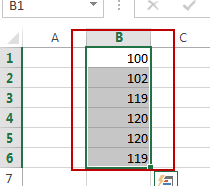Step2: go to DATA tab, click Advanced command under Sort & Filter group. And the Advanced Filter dialog will open.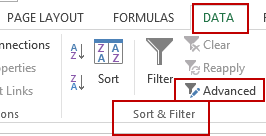Step3: select Copy to another location radio button in the Action section. And select one blank cell where you want to paste unique values in Copy to text box.  Checked Unique records only . click Ok button.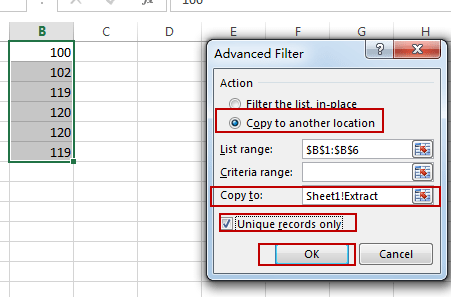Step4: you would see that a list of unique values is generated.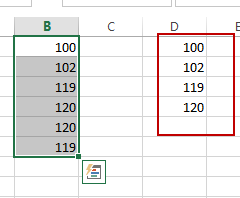Stet5: go to one blank cell and insert the following formula and hit enter, you would get the number of unique values for your range of cells.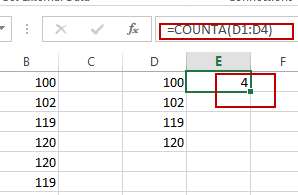## Count Unique Values using Formula

You can also use an Excel formula to count the number of unique values in a range of cells, you can use the COUNTIF function in combination with SUMPRODUCT function to create a formula. Just like this:

`=SUMPRODUCT(1/COUNTIF(B1:B6,B1:B6))`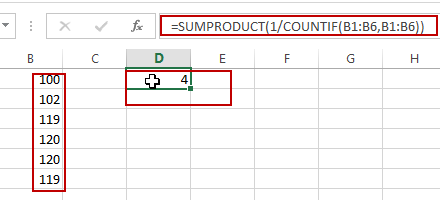Note: you can also use another Array formula based on the SUM function and the CountIF function to achieve the same result. Like this:

`=SUM(1/COUNTIF(B1:B6,B1:B6))`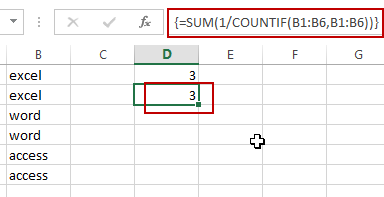You need to press Ctrl + Shift + Enter to change the above common formula as array formula.

### You might also like:

Sidebar